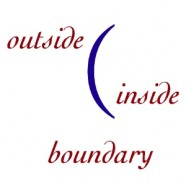# Iconic PrinciplesT he general principles of iconic mathematics can be applied to many mathematical domains. Several new fields of mathematics (such as knot theory, category theory and brane theory) rely on iconic techniques. Several old fields of mathematics (such as basic algebra, vector algebra and geometry) incorporate both iconic and symbolic perspectives.

The Image shows a fundamental property of Boundary Math: a spatial distinction consists of three inseparable components.

The roots of iconic mathematics are deep in history, since almost all math prior to the invention of symbolic techniques in the 16th century was either linguistic or iconic in nature. The first formalization of iconic techniques occurred in the mid-19th century, with Venn diagrams and Pierce’s Existential graphs.

Spencer-Brown’s mathematical text Laws of Form summarizes the formal principles of iconic systems. Iconic mathematics incorporates both the participant and the void into its formal structure. This makes iconic formal theorems and proof techniques fundamentally different than symbolic techniques.

This short article, The Mathematics of Boundaries: A Beginning describes the construction of both arithmetic and logic out of empty space. Here is the abstract.

The intuitive properties of configurations of planar non-overlapping closed curves (boundaries) are presented as a pure boundary mathematics. The mathematics, which is not incorporated in any existing formalism, is constructed from first principles, that is, from empty space. When formulated as pattern-equations, boundary algebras map to elementary logic and to integer arithmetic.

### General Principles of Iconic Mathematics

1.  Mathematics is the experience of abstraction.

2.  Experience is not a recording.  Representation is not reality.

3.  The void cannot be represented.

4.  Space requires participation.  To participate is to partition space, to construct a boundary.

5.  Boundaries both separate and connect.

6.  Boundaries identify an intentional construction.

7.  Representation and meaning are different sides of the same boundary.

8.  Our body is our interface.

### Mathematical Principles

9.  Operators, invariants, and identities can be embedded in space.

10. Multiplicity is generated by observation.

11. Commutativity is embedded in space, ordering is embedded in time.  Virtual entities are asynchronous parallel processes.

12. Associativity is the choice of the participant.  Entities are autonomous.

13.  Entities are both singular and plural in form, depending upon the construction of the participant.  Entities with the same name are the same entity.

14.  That which is common to every entity in a space is common to the space itself, forming the ground of the space.

15. Touching spaces are in pervasive contact (Cartesian product).

16. Crossing a boundary inverts a space.  Inversion unites partitioned spaces.

17. Normalized spaces are those equivalent to the void.  They can support arbitrary grounds.

### Canons of Formal Symbol Systems

The canons of formal symbol systems are those conventions that guide our agreement about the semantics, or meaning, and the syntax, or representation, of formal systems. These canons define the meaning of formality. An error within a formal system is defined as a violation of one of these canons. The canons provide a complete set of rules to motivate mathematical behavior. The following list is loosely paraphrased from Spencer-Brown’s Laws of Form, one of the few mathematics texts that addresses the assumptions underlying the use of formal symbol systems.

### Canons

#### Intention

• Permissible transformations are specified by rules.

• New rules or conventions must be mutually agreed upon.

• What is not explicitly permitted is forbidden.

• Formal constraints are by choice.

#### Reference

• The representation of a concept is not inherently confusing.

• Symbols represent concepts that can be understood.

• Contraction of reference: Representations may be abbreviated to any extent that is understood.

• Expansion of reference: New representations may be introduced without limit, provided that confusion is avoided.

#### Substitution

• In any expression, any arrangement can be exchanged for an equivalent arrangement.

• Equivalent intentions are expressed by equivalent symbols.

#### Simplification/Transformation

• Following the rules does not change the meanings.

• Meaning is preserved when steps are taken.

• Symbols may be manipulated within the rules without altering their assigned interpretations.

#### Relevance

• Universal properties within a system need not be indicated.

• A mutually agreed upon interpretative context is assumed.

• If a property is common to every indication, it need not be indicated.

#### Demonstration

• A demonstration rests in a finite number of steps.

#### Dominance

• Meaning is indicated overtly in the form of expressions.

• The form of an expression indicates the choice of a meaning.

#### Transmission

• When a value of a variable oscillates (by ambiguity or by choice), the interpretative context of the variable is either transparent or opaque to that oscillation.

### Axioms and Theorems of Representation

#### Axioms

• The interpretation of a symbol does not change independently of the interpreter.

• Reinterpretation denies the original interpretation.

#### Equivalence of Transformation

• The product of transformation steps is independent of the choice or sequence of steps, given a consistent result.

• All transformation paths leading to the same result are equivalent.

#### Theorems of Representation

• Form: An expression is represented by its form.

• Content: The value of an expression is the value of its form.

• Agreement: Simplification is unique.

• Distinction: Rules specify permissible transformation steps.

• Steps do not change the meaning of an expression, although they do change the form.

• If the value of two expressions differ, then steps taken on the two expressions maintain that difference.

#### Procedural Theorems

• Identity: Expressions with identical forms represent identical values.

• Value: Expressions with the same value can be identified.

• Consequence: Expressions equivalent in value to a given expression are equivalent to each other.

#### Connective Theorems

• Invariance: If a distinction distinguishes equivalent expressions, it is not a distinction.

• Variance: If different expressions each contain the same arrangement, then this arrangement can be communicated to the interpretative context of the expressions.